Disclaimer: This is an example of a student written essay.

Any opinions, findings, conclusions or recommendations expressed in this material are those of the authors and do not necessarily reflect the views of UKEssays.com.

# Number of Folds in Paper: Thickness of Earth to Sun

 ✅ Paper Type: Free Essay ✅ Subject: Mathematics ✅ Wordcount: 2735 words ✅ Published: 8th Sep 2017

Calculating the number of folds and the hypothetical size of a piece of paper so that its thickness equates to the distance from the Earth to Saturn.

As a physics student doing the option on astrophysics, I have become very interested in the immensity of the universe. I decided it would be intriguing to combine it with my personal hobby of doing origami which helps me to relieve stress. As a result, I investigated the amount of times a piece of paper would need to be folded in half so that its thickness achieves a length which equates to the distance from the Earth to Saturn. I have chosen the planet Saturn because it is one of the biggest planets that can be observed by the naked eye and I have been able to see it last summer from the top of a high hill in La Pedriza in the Guadarrama mountain range near my hometown (Collado Villalba, Madrid, Spain).

Popular claims suggested that it is impossible to fold a piece of paper in half more than seven times no matter its size or thickness.  Previous mathematicians have worked out the number of folds required to reach the moon from the Earth which was worked out to be 42 folds using a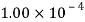m thick paper. The size of the paper however, was not found. In theory, the average folds for a normal size A4 (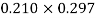m) paper is seven.

This is an interesting topic because it is incredible how, by exponential growth, a miniature of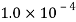m thick like a piece of paper can be folded in half to reach the distance of the planet Saturn.

Britney Gallivan was able to fold a piece of toilet paper of 1200 meters a number of 12 folds. She derived two mathematical expressions based on geometrical sequences, taking into consideration the amount of paper lost in every fold. These formulas make it possible to calculate the hypothetical length L and width W of a piece of paper that would be folded n times to equate the distance from the Earth to Saturn.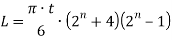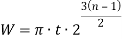Gallivan established some rules that would need to be followed when folding a sheet of paper in half:

1. A single rectangular sheet of paper of any size and uniform thickness can be used.
2. The fold line has to be in the same direction each time.
3. The folding process must not tear the paper.
4. When folded in half, the portions of the inner layers which face one another must almost touch one another.
5. The average thickness or structure of material of paper must remain unaffected by the folding process.
6. A fold is considered complete if portions of all layers lie in one straight line.

Hence, the length L of the paper influences the number of times it can be folded in half.

Hypothesis – The distance from the Earth to Saturn will be obtained by folding a piece of paper ≥50 times with a hypothetically large enough paper.

This exploration used exponential growth and logarithms in order to find out the number of folds required to reach Saturn. Before any calculation was done, it was indispensable to collect all the data required for the investigation. All the values were used were in the international system of units (metres) and standard form in order to keep the exploration standardized.

Taking into consideration the elliptical orbits of the planets, sometimes they are closer to Earth and sometimes they are further away. Therefore, the value used during this mathematical exploration was the mean value of when they are the furthest apart and the closest together. Astronomical units (AU) are the standard unit measure used when dealing with distances within the Solar System. 1 AU is equal to the distance from the Earth to the Sun which is equivalent to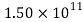m.

Distance from the Earth to Saturn when closer together – 8.00 AU

Distance from the Earth to Saturn when further apart – 11.0 AU3

Mean distance from the Earth to Saturn –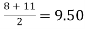AU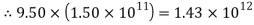m

The thickness of a normal A4 paper (0.210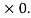297m) was calculated by taking a measurement of an office pack of 500 pages (80gsm) which was found to be about 0.05m. The thickness of each individual page is calculated by dividing the total thickness (0.05m) by the amount of sheets (500); giving a result ofm.

Whenever a paper is folded in half, the number of layers is doubled so the thickness increases by two. When there is only one layer of paper (not folded), its thickness ism. Once it is folded in half for the first time, its thickness will be multiplied by 2 hence,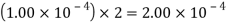m

Folding it one more time means multiplying it by two again,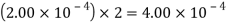m

Thus, an expression can be established, showing the exponential growth;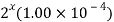The expression can be represented in a graph to illustrate graphically the exponential growth because of folding.

Graph 1: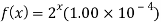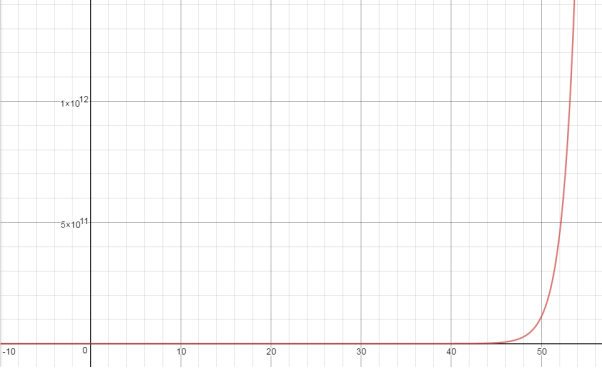From Graph 1, it is possible to visualise how, something that seems unrealistic like folding a sheet of paper to reach Saturn becomes possible. The graph also illustrates how rapidly exponential growth occurs.

Since the expression needs to be equal to the distance from the Earth to Saturn to work out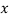(the number of folds), an equation to findcan be solved: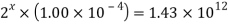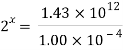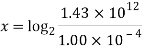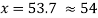Folds

To find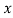, the rules of logarithms were put in place due to the exponential nature of the equation. The answer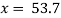has been rounded up to 54 because it is not possible to have a half fold.

Gallivan derived the following formula for the minimum length of a piece of paper of thickness t to be folded n times in a single directionTo prove this formula, it is neccesary to understand that after each fold, some part of the paper is lost and becomes a rounded edge. I folded an A4 sheet of paper seven times in order to illustrate this:

As you can see from the picture, there is a rounded edge on the side which is paper being lost and is not contributing to the real thickness but just joining the layers. The curved portion becomes bigger in correlation with the number of folds and begins to take a greater area of the volume of the paper.

At the first fold, a semicircle of radius t (thickness) is formed, which has a perimeter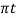. Thus,units of the paper are being used in the fold.  A paper smaller than this cannot be folded since there is not enough paper to form the fold. After the fold, there is a two-layer sheet of paper with a thickness of 2t. Another fold results in folding the second layer over the first layer. The second layer has a radius of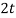, so it uses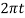units of paper. The total amount of paper used by the second fold, for both layers, is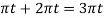resulting in a four-layer piece of paper.

The ith fold begings with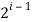layers, and folding the jth layer uses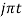units of paper. Hence, the total length of paper used for the ith fold is given by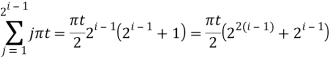Therefore, to obtain the total length of paper required for n number of folds, sum this over i from 1 to n, which gives Gallivan’s formula: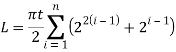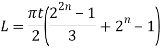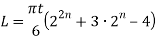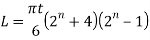The thickness can be substituted into t and the number of folds can be substituted into n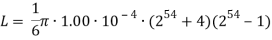which gives L to be equal to 1.70-1028m.

The other expression proposed by Gallivan can be used to calculate the width of the paper.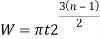If the length lost in the radii of earlier folds is not considered, the length lost must be considered in the last fold. At the final fold n, the side of the square must be at least equal to the length lost in the final fold which is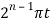(amount of length lost in each fold. Taking into consideration that the total area of the sheet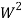(area = nb of sheets in penultimate step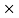area of square in penultimate step) is preserved, Gallivan’s equation can be derived: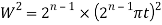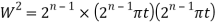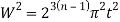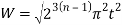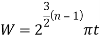Again, the thickness and number of folds can be substituted and an answer for W can be found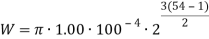giving W to be 2.69-1020m.

In conclusion, the initial hypothesis was right since the number of folds was 54 which is, indeed, greater than 50. The hypothetical paper that could, in theory, be folded 54 times so that its thickness equates the distance from the Earth to Saturn of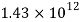m would be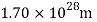long and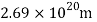wide (taking into consideration that its thickness would be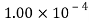m). The dimensions of this paper would be bigger than the actual distance from the Earth to Saturn so, unfortunately, we do not have a paper that big that would allow us to reach to Saturn just by folding it in half.

This mathematical exploration used logarithms to find out the number of folds needed to reach Saturn with a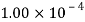m thick paper. However, the dimensions of this sheet of paper would be too big and hence, impossible to find in the Earth’s surface. Nevertheless, the exploration could have looked at using a thinner piece of paper to see if its dimensions would have been smaller and perhaps, we would have been able to find it in the surface of the Earth and we would have been able to reach Saturn.

References

Astronomy, S. (2012). How Far away is Saturn? [Online] Space.com. Available at: http://www.space.com/18477-how-far-away-is-saturn.html [Accessed 16 Jan. 2017].

IFLScience. (2016). Fold A Piece of Paper in Half 103 Times and It Will Be As Thick As the UNIVERSE. [Online] Available at: http://www.iflscience.com/space/fold-piece-paper-half-103-times-and-it-will-be-thick-universe/ [Accessed 16 Jan. 2017].

Pomonahistorical.org. (2002). Folding Paper in Half Twelve Times. [Online] Available at: http://pomonahistorical.org/12times.htm [Accessed 16 Jan. 2017].

 IFLScience. (2016). Fold A Piece of Paper in Half 103 Times and It Will Be As Thick As the UNIVERSE. [online] Available at: http://www.iflscience.com/space/fold-piece-paper-half-103-times-and-it-will-be-thick-universe/ [Accessed 16 Jan. 2017].

Pomonahistorical.org. (2002). Folding Paper in Half Twelve Times. [online] Available at: http://pomonahistorical.org/12times.htm [Accessed 16 Jan. 2017].

 Astronomy, S. (2012). How Far away is Saturn? [online] Space.com. Available at: http://www.space.com/18477-how-far-away-is-saturn.html [Accessed 16 Jan. 2017].

View all

## DMCA / Removal Request

If you are the original writer of this essay and no longer wish to have your work published on UKEssays.com then please:

Related Services

Prices from

S\$203

Approximate costs for:

• 1000 words
• 7 day deliveryHumanity University

Dedicated to your worth and value as a human being!

Related Lectures